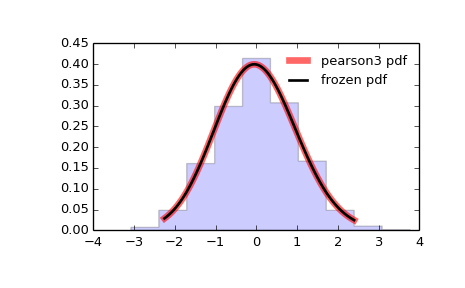# scipy.stats.pearson3¶

scipy.stats.pearson3 = <scipy.stats._continuous_distns.pearson3_gen object at 0x45083b2c>[source]

A pearson type III continuous random variable.

As an instance of the rv_continuous class, pearson3 object inherits from it a collection of generic methods (see below for the full list), and completes them with details specific for this particular distribution.

Notes

The probability density function for pearson3 is:

pearson3.pdf(x, skew) = abs(beta) / gamma(alpha) *
(beta * (x - zeta))**(alpha - 1) * exp(-beta*(x - zeta))


where:

beta = 2 / (skew * stddev)
alpha = (stddev * beta)**2
zeta = loc - alpha / beta


pearson3 takes skew as a shape parameter.

The probability density above is defined in the “standardized” form. To shift and/or scale the distribution use the loc and scale parameters. Specifically, pearson3.pdf(x, skew, loc, scale) is identically equivalent to pearson3.pdf(y, skew) / scale with y = (x - loc) / scale.

References

R.W. Vogel and D.E. McMartin, “Probability Plot Goodness-of-Fit and Skewness Estimation Procedures for the Pearson Type 3 Distribution”, Water Resources Research, Vol.27, 3149-3158 (1991).

L.R. Salvosa, “Tables of Pearson’s Type III Function”, Ann. Math. Statist., Vol.1, 191-198 (1930).

“Using Modern Computing Tools to Fit the Pearson Type III Distribution to Aviation Loads Data”, Office of Aviation Research (2003).

Examples

>>> from scipy.stats import pearson3
>>> import matplotlib.pyplot as plt
>>> fig, ax = plt.subplots(1, 1)


Calculate a few first moments:

>>> skew = 0.1
>>> mean, var, skew, kurt = pearson3.stats(skew, moments='mvsk')


Display the probability density function (pdf):

>>> x = np.linspace(pearson3.ppf(0.01, skew),
...                 pearson3.ppf(0.99, skew), 100)
>>> ax.plot(x, pearson3.pdf(x, skew),
...        'r-', lw=5, alpha=0.6, label='pearson3 pdf')


Alternatively, the distribution object can be called (as a function) to fix the shape, location and scale parameters. This returns a “frozen” RV object holding the given parameters fixed.

Freeze the distribution and display the frozen pdf:

>>> rv = pearson3(skew)
>>> ax.plot(x, rv.pdf(x), 'k-', lw=2, label='frozen pdf')


Check accuracy of cdf and ppf:

>>> vals = pearson3.ppf([0.001, 0.5, 0.999], skew)
>>> np.allclose([0.001, 0.5, 0.999], pearson3.cdf(vals, skew))
True


Generate random numbers:

>>> r = pearson3.rvs(skew, size=1000)


And compare the histogram:

>>> ax.hist(r, normed=True, histtype='stepfilled', alpha=0.2)
>>> ax.legend(loc='best', frameon=False)
>>> plt.show()Methods

 rvs(skew, loc=0, scale=1, size=1, random_state=None) Random variates. pdf(x, skew, loc=0, scale=1) Probability density function. logpdf(x, skew, loc=0, scale=1) Log of the probability density function. cdf(x, skew, loc=0, scale=1) Cumulative density function. logcdf(x, skew, loc=0, scale=1) Log of the cumulative density function. sf(x, skew, loc=0, scale=1) Survival function (1 - cdf — sometimes more accurate). logsf(x, skew, loc=0, scale=1) Log of the survival function. ppf(q, skew, loc=0, scale=1) Percent point function (inverse of cdf — percentiles). isf(q, skew, loc=0, scale=1) Inverse survival function (inverse of sf). moment(n, skew, loc=0, scale=1) Non-central moment of order n stats(skew, loc=0, scale=1, moments='mv') Mean(‘m’), variance(‘v’), skew(‘s’), and/or kurtosis(‘k’). entropy(skew, loc=0, scale=1) (Differential) entropy of the RV. fit(data, skew, loc=0, scale=1) Parameter estimates for generic data. expect(func, skew, loc=0, scale=1, lb=None, ub=None, conditional=False, **kwds) Expected value of a function (of one argument) with respect to the distribution. median(skew, loc=0, scale=1) Median of the distribution. mean(skew, loc=0, scale=1) Mean of the distribution. var(skew, loc=0, scale=1) Variance of the distribution. std(skew, loc=0, scale=1) Standard deviation of the distribution. interval(alpha, skew, loc=0, scale=1) Endpoints of the range that contains alpha percent of the distribution

#### Previous topic

scipy.stats.pareto

#### Next topic

scipy.stats.powerlaw